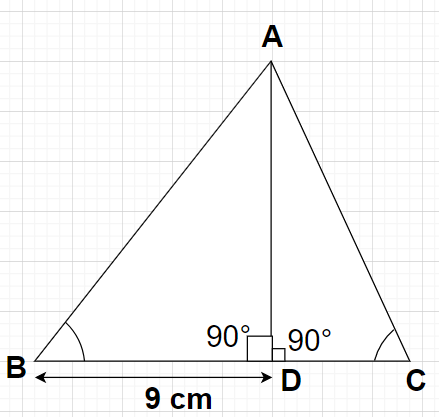QuestionAnswers

# In triangle ABC, AD is perpendicular to BC, $\sin {\text{B}} = 0.8$, BD = 9 cm and $\tan {\text{C}} = 1$. Find the length of AB.Hint-Hint- Here, we will proceed by drawing the figure corresponding to the problem statement and then, we will use the identity to find cosB which will eventually give the value of the length AB.In $\vartriangle$ABC, $\sin {\text{B}} = 0.8$, BD = 9 cm and $\tan {\text{C}} = 1$
AD is the perpendicular drawn from point A to side BC of the triangle ABC which will divide the triangle ABC into two right angled triangles with right angle at vertex D.
As we know that ${\left( {\sin \theta } \right)^2} + {\left( {\cos \theta } \right)^2} = 1$
For angle B using the above identity, we can write
${\left( {\sin {\text{B}}} \right)^2} + {\left( {\cos {\text{B}}} \right)^2} = 1$
By substituting $\sin {\text{B}} = 0.8$ in the above equation, we get
$\Rightarrow {\left( {0.8} \right)^2} + {\left( {\cos {\text{B}}} \right)^2} = 1 \\ \Rightarrow {\left( {\cos {\text{B}}} \right)^2} = 1 - {\left( {0.8} \right)^2} \\ \Rightarrow {\left( {\cos {\text{B}}} \right)^2} = 1 - 0.64 \\ \Rightarrow {\left( {\cos {\text{B}}} \right)^2} = 0.36 \\ \Rightarrow \cos {\text{B}} = \pm \sqrt {0.36} \\ \Rightarrow \cos {\text{B}} = \pm 0.6 \\$
In any right angled triangle, according to the definition of cosine trigonometric function, we have
$\cos \theta = \dfrac{{{\text{Base}}}}{{{\text{Hypotenuse}}}}$
Using the above formula, we can write
$\cos {\text{B}} = \dfrac{{{\text{BD}}}}{{{\text{AB}}}} \\ \Rightarrow {\text{AB}} = \dfrac{{{\text{BD}}}}{{\cos {\text{B}}}} \\$
By substituting BD = 9 cm and $\cos {\text{B}} = \pm 0.6$ in the above equation, we get
$\Rightarrow {\text{AB}} = \dfrac{{\text{9}}}{{ \pm 0.6}}$
Here, we will be considering only the positive value of cosine of angle B because the length of the side of any triangle is always positive. So, cosB = -0.6 is neglected.
$\Rightarrow {\text{AB}} = \dfrac{{\text{9}}}{{0.6}} = \dfrac{{90}}{6} \\ \Rightarrow {\text{AB}} = 15{\text{ cm}} \\$
Therefore, the length of side AB of the triangle is 15 cm.

Note- In this particular problem, we can also find the length AD using the Pythagoras Theorem in triangle ABD i.e., ${\left( {{\text{Hypotenuse}}} \right)^2} = {\left( {{\text{Perpendicular}}} \right)^2} + {\left( {{\text{Base}}} \right)^2} \Rightarrow {\left( {{\text{AB}}} \right)^2} = {\left( {{\text{AD}}} \right)^2} + {\left( {{\text{BD}}} \right)^2}$. Also, using $\tan {\text{C}} = \dfrac{{{\text{AD}}}}{{{\text{CD}}}}$ we will get the length CD and for the length AC we will use Pythagoras Theorem in triangle ACD i.e., ${\left( {{\text{AC}}} \right)^2} = {\left( {{\text{AD}}} \right)^2} + {\left( {{\text{CD}}} \right)^2}$.

View Notes
Sin 120Value of Sin 180CBSE Class 11 Maths FormulasCBSE Class 7 Maths Chapter 6 - Triangle and Its Properties FormulasRight Angled Triangle ConstructionsGroup Theory in MathematicsRelation Between Mean Median and ModeTable 11 to 30CBSE Class 6 Maths Chapter 11 - Algebra FormulasSin Cos FormulaImportant Questions for CBSE Class 11 Maths Chapter 3 - Trigonometric FunctionsImportant Questions for CBSE Class 11 Maths Chapter 12 - Introduction to Three Dimensional GeometryImportant Questions for CBSE Class 11 Accountancy Chapter 1 - Introduction To AccountingImportant Questions for CBSE Class 11 Biology Chapter 11 - Transport in PlantsImportant Questions for CBSE Class 11 MathsImportant Questions for CBSE Class 11 Maths Chapter 11 - Conic SectionsImportant Questions for CBSE Class 11 Maths Chapter 15 - StatisticsImportant Questions for CBSE Class 8 Maths Chapter 15 - Introduction to GraphsImportant Questions for CBSE Class 10 Maths Chapter 8 - Introduction to TrigonometryImportant Questions for CBSE Class 11 Biology Chapter 14 - Respiration in PlantsHindi B Class 10 CBSE Question Paper 2007Hindi B Class 10 CBSE Question Paper 2015Hindi B Class 10 CBSE Question Paper 2010Hindi B Class 10 CBSE Question Paper 2012Hindi B Class 10 CBSE Question Paper 2009Hindi B Class 10 CBSE Question Paper 2008Hindi B Class 10 CBSE Question Paper 2014Hindi B Class 10 CBSE Question Paper 2013Hindi B Class 10 CBSE Question Paper 2016CBSE Class 10 Hindi Course B Question Paper 2019 - Free PDFRS Aggarwal Class 10 Solutions - Trigonometric RatiosRD Sharma Solutions for Class 10 Maths Chapter 5 - Trigonometric RatiosRD Sharma Class 11 Maths Solutions Chapter 7 - Trigonometric Ratios of Compound AnglesNCERT Solutions for Class 11 Maths Chapter 3 Trigonometric Functions in HindiNCERT Solutions for Class 11 Maths Chapter 12 Introduction to Three Dimensional Geometry in HindiRS Aggarwal Class 10 Solutions - Trigonometric Ratios of Complementary AnglesRD Sharma Class 11 Maths Solutions Chapter 11 - Trigonometric EquationsRD Sharma Class 11 Maths Solutions Chapter 9 - Trigonometric Ratios of Multiple and Sub-multiple AnglesNCERT Solutions for Class 7 Maths Chapter 6 The Triangle and Its Properties In HindiRS Aggarwal Class 9 Solutions Chapter-9 Congruence of Triangles and Inequalities in a Triangle